# SECTION 4 5 Graphs of Sine Cosine Functions

• Slides: 32
Download presentationSECTION 4. 5 Graphs of Sine & Cosine Functions MA. PC. 4. 5 2000 Define and graph trigonometric functions (i. e. , sine, cosine, tangent, cosecant, cotangent). MA. PC. 4. 6 2000 Find domain, range, intercepts, periods, amplitudes, and asymptotes of trigonometric functions. MA. PC. 4. 7 2000 Draw and analyze graphs of translations of trigonometric functions, including period, amplitude, and phase shift.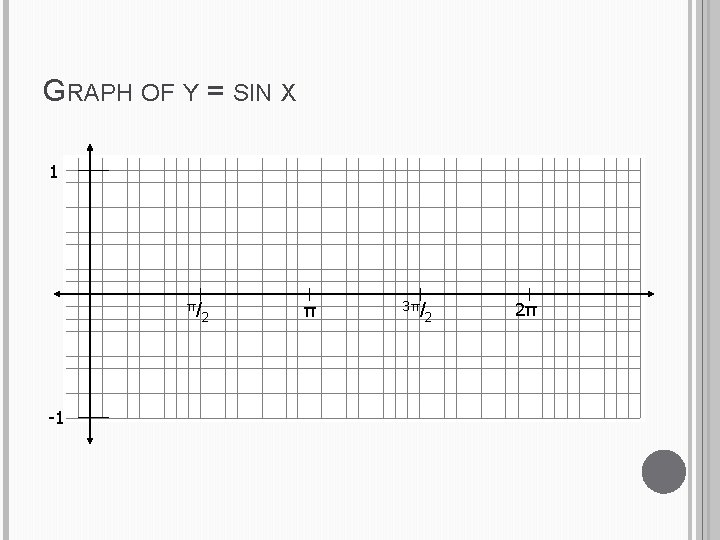GRAPH OF Y = SIN X 1 π/ -1 2 π 3π/ 2 2πDomain: all real numbers Range: -1<y<1PERIODIC FUNCTION sin(t+2 )=sin t cos(t+2 )=cos t The sine and cosine functions are periodic functions and have period 2GRAPH OF Y = COS X 1 π/ -1 2 π 3π/ 2 2π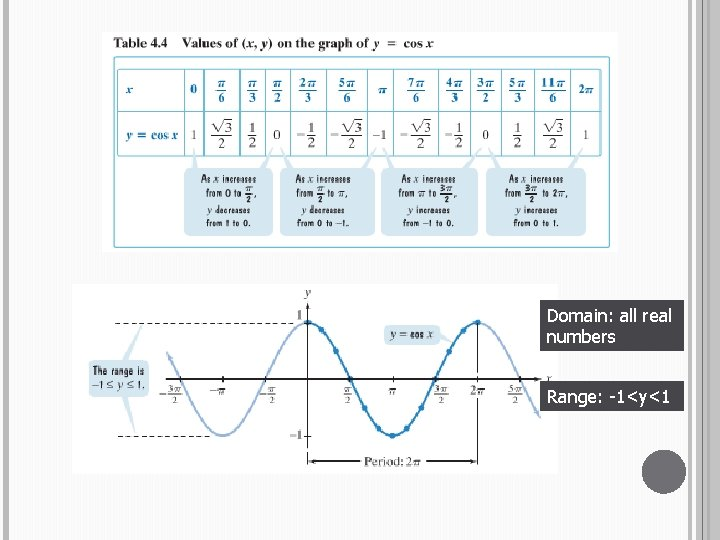Domain: all real numbers Range: -1<y<1AMPLITUDES AND PERIODS Given the graph of y=Asin Bx Amplitude = |A| = distance up or down from center Period = 2 /B = time taken to repeat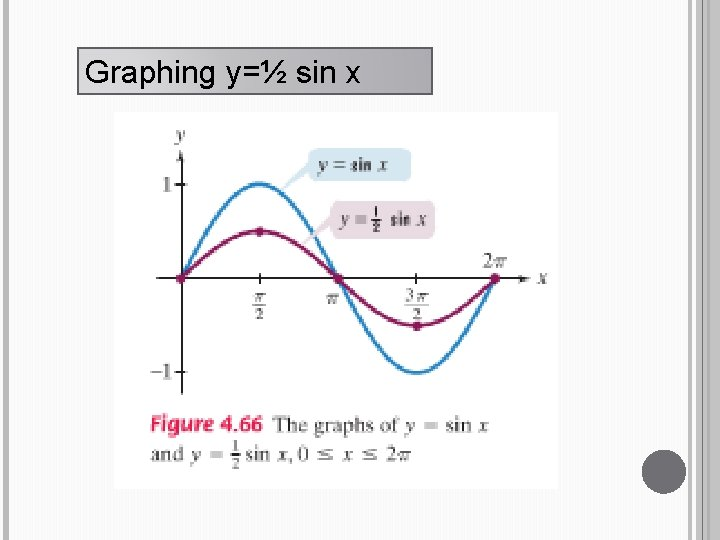Graphing y=½ sin x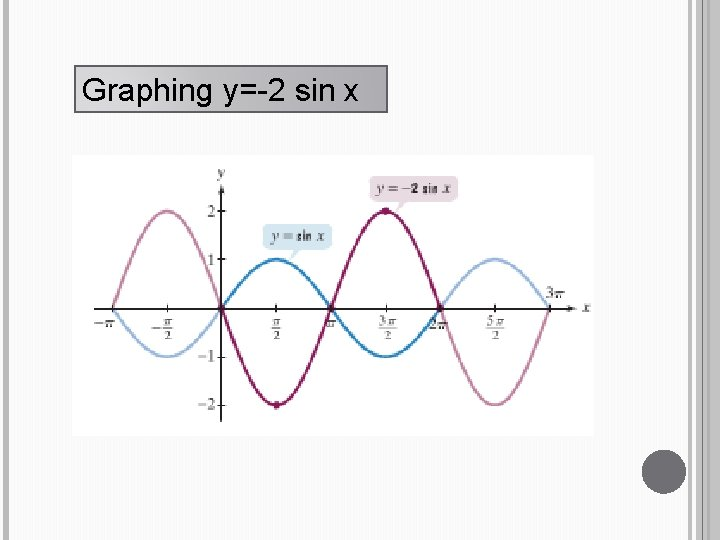Graphing y=-2 sin xGraphing y=3 sin 2 x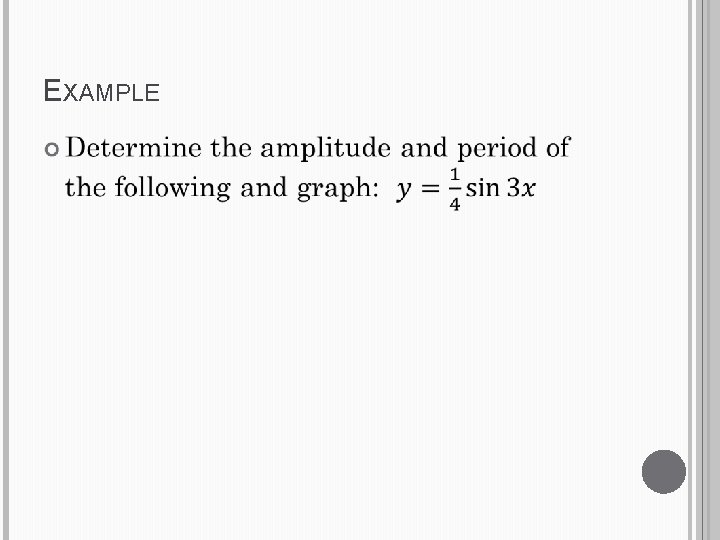EXAMPLE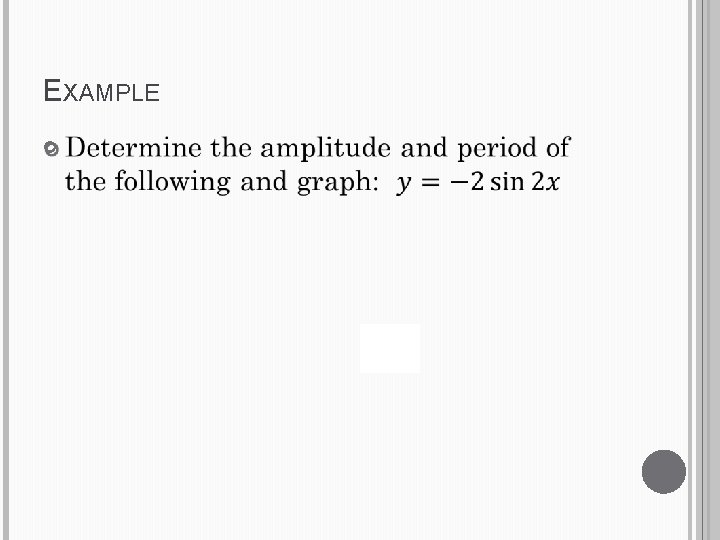EXAMPLE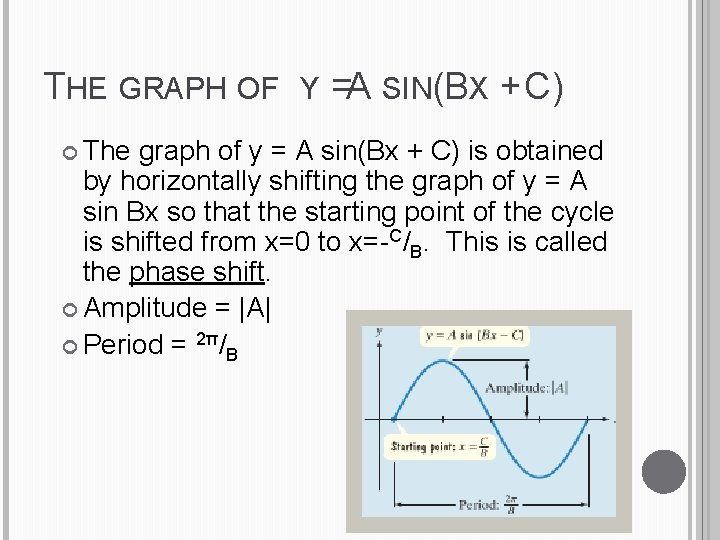THE GRAPH OF Y = A SIN(BX + C) The graph of y = A sin(Bx + C) is obtained by horizontally shifting the graph of y = A sin Bx so that the starting point of the cycle is shifted from x=0 to x=-C/B. This is called the phase shift. Amplitude = |A| Period = 2π/B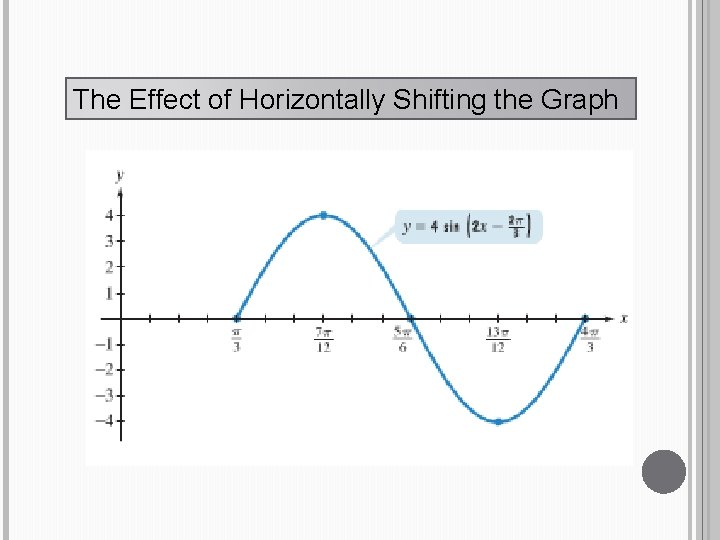The Effect of Horizontally Shifting the Graph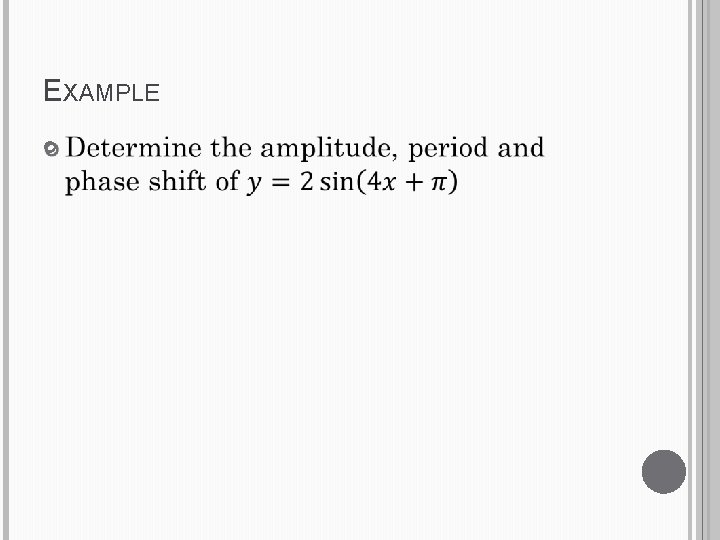EXAMPLEEXAMPLECosine with a Different Amplitude and Period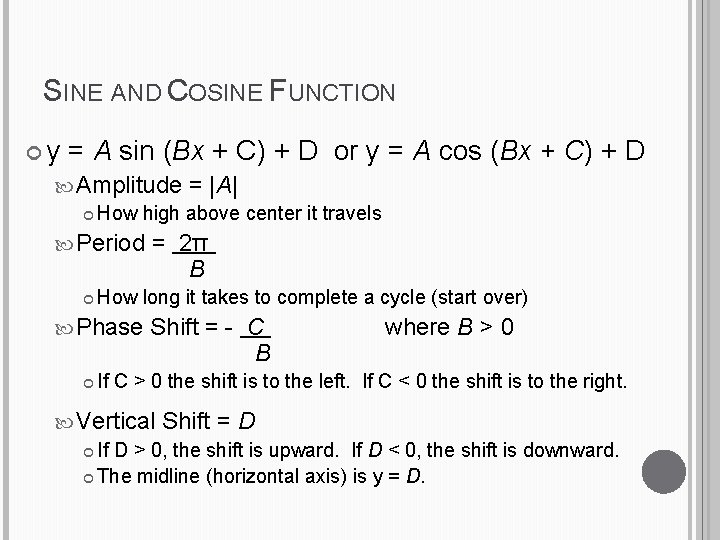SINE AND COSINE FUNCTION y = A sin (Bx + C) + D or y = A cos (Bx + C) + D Amplitude = |A| How high above center it travels Period = 2π B How long it takes to complete a cycle (start over) Phase Shift = - C B where B > 0 If C > 0 the shift is to the left. If C < 0 the shift is to the right. Vertical Shift = D If D > 0, the shift is upward. If D < 0, the shift is downward. The midline (horizontal axis) is y = D.HOMEWORK Page 533: 20 -56 every four Do NOT graph. Just identify the values.SECTION 4. 5 Graphs of Sine & Cosine Functions MA. PC. 4. 5 2000 Define and graph trigonometric functions (i. e. , sine, cosine, tangent, cosecant, cotangent). MA. PC. 4. 6 2000 Find domain, range, intercepts, periods, amplitudes, and asymptotes of trigonometric functions. MA. PC. 4. 7 2000 Draw and analyze graphs of translations of trigonometric functions, including period, amplitude, and phase shift.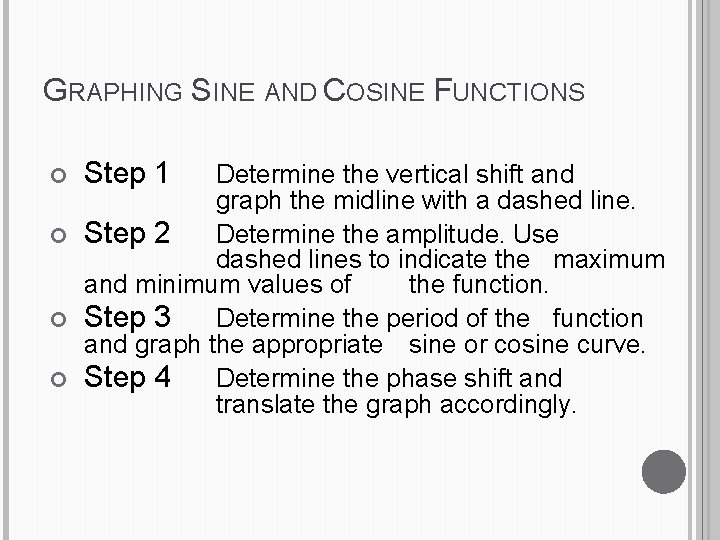GRAPHING SINE AND COSINE FUNCTIONS Step 1 Determine the vertical shift and graph the midline with a dashed line. Step 2 Determine the amplitude. Use dashed lines to indicate the maximum and minimum values of the function. Step 3 Determine the period of the function and graph the appropriate sine or cosine curve. Step 4 Determine the phase shift and translate the graph accordingly.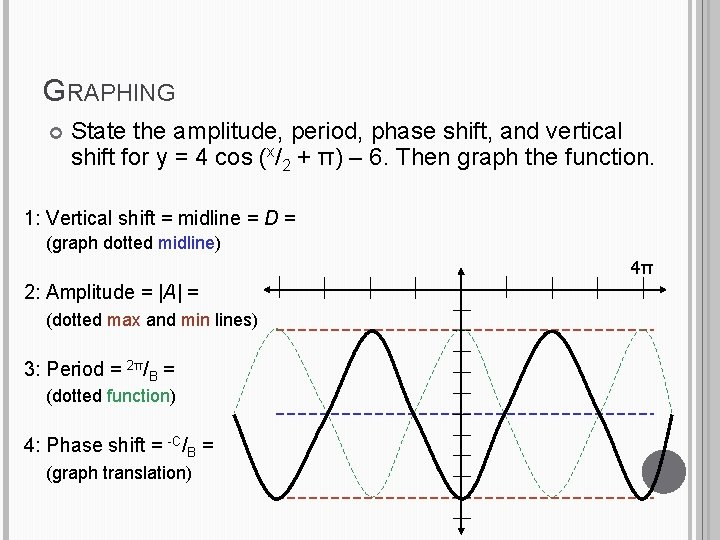GRAPHING State the amplitude, period, phase shift, and vertical shift for y = 4 cos (x/2 + π) – 6. Then graph the function. 1: Vertical shift = midline = D = (graph dotted midline) 4π 2: Amplitude = |A| = (dotted max and min lines) 3: Period = 2π/B = (dotted function) 4: Phase shift = -C/B = (graph translation)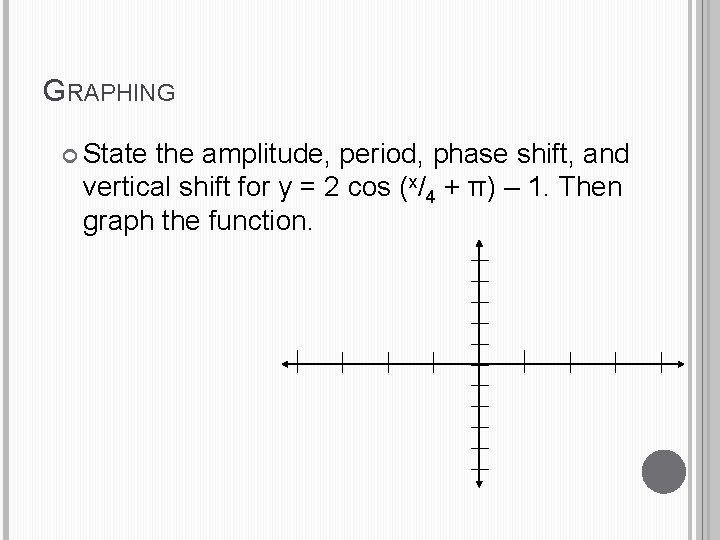GRAPHING State the amplitude, period, phase shift, and vertical shift for y = 2 cos (x/4 + π) – 1. Then graph the function.MODELING PERIODIC BEHAVIOR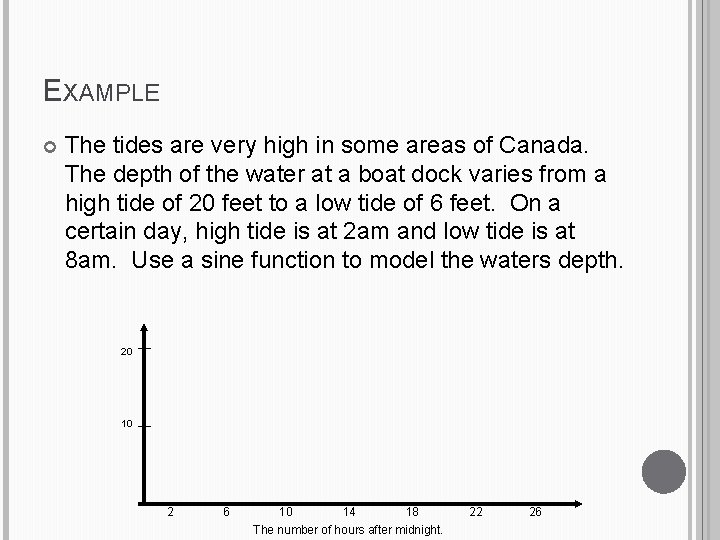EXAMPLE The tides are very high in some areas of Canada. The depth of the water at a boat dock varies from a high tide of 20 feet to a low tide of 6 feet. On a certain day, high tide is at 2 am and low tide is at 8 am. Use a sine function to model the waters depth. 20 10 2 6 10 14 18 22 26 The number of hours after midnight.Simple Harmonic MotionSIMPLE HARMONIC MOTION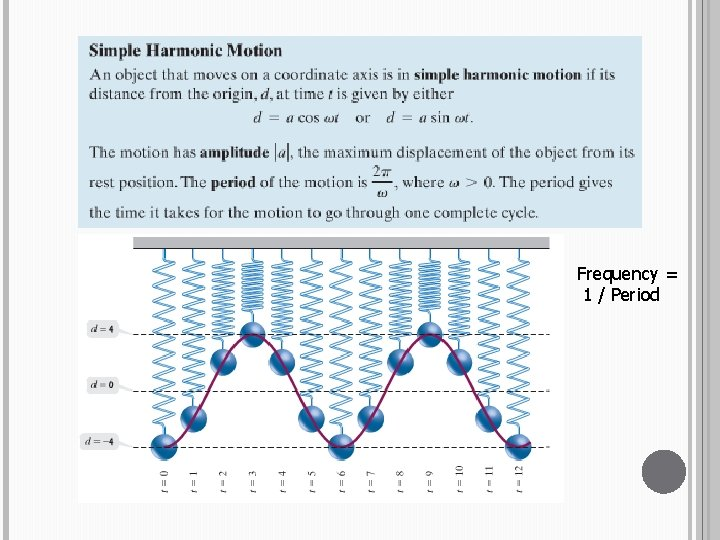Frequency = 1 / Period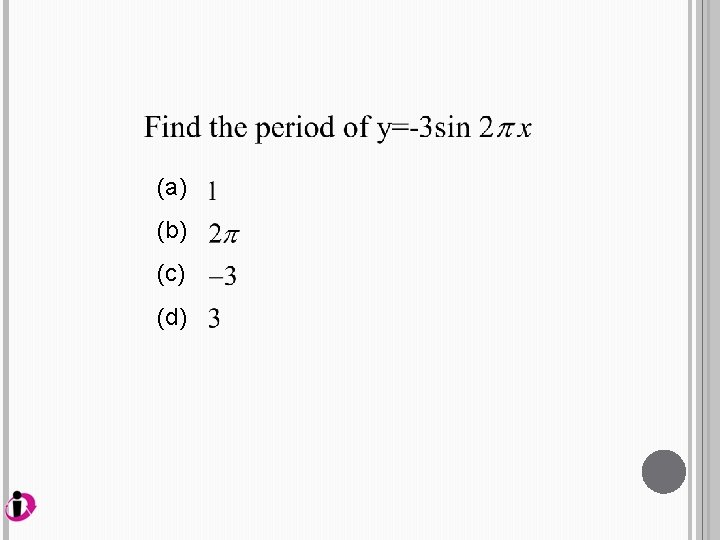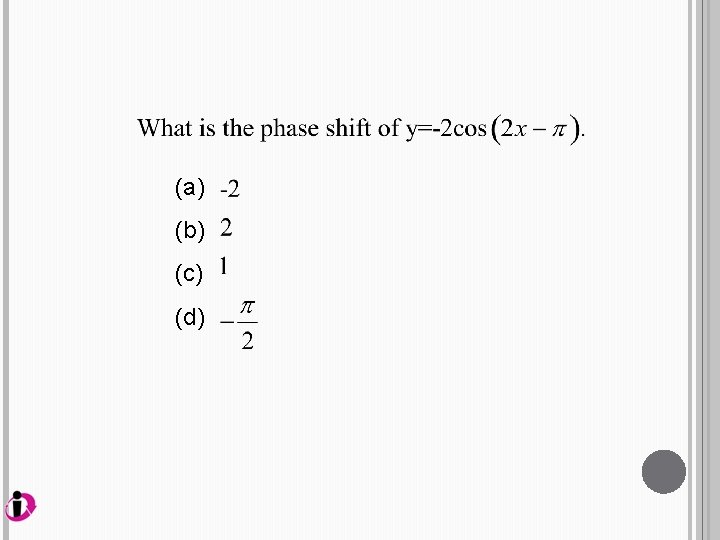HOMEWORK Page 533: 58 -64 even, 85